# Halves - Definition with Examples

The Complete K-5 Math Learning Program Built for Your Child

• 40 Million Kids

Loved by kids and parent worldwide

• 50,000 Schools

Trusted by teachers across schools

• Comprehensive Curriculum

Aligned to Common Core

## What is Halves?

Splitting a whole thing into two equal parts gives a half.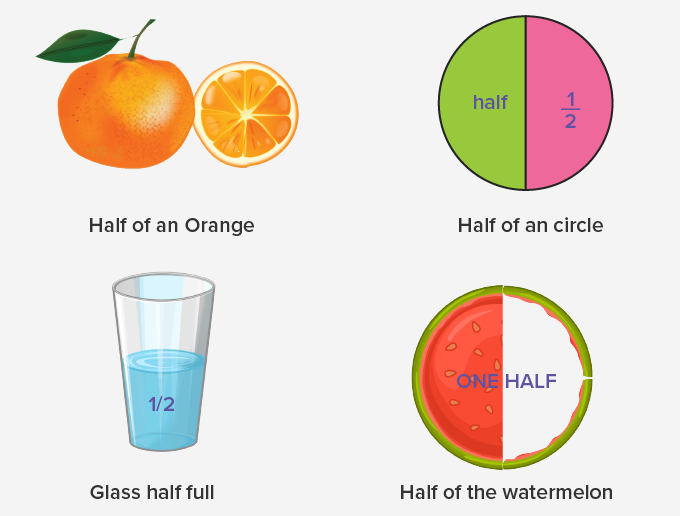## Halves of various shapes

In the given images, the colored portions in each shape represent halves.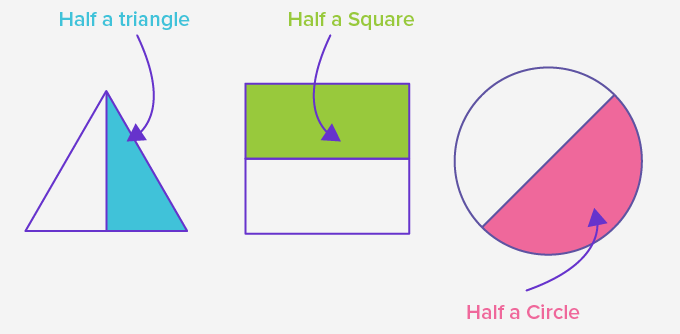Representing half using fractions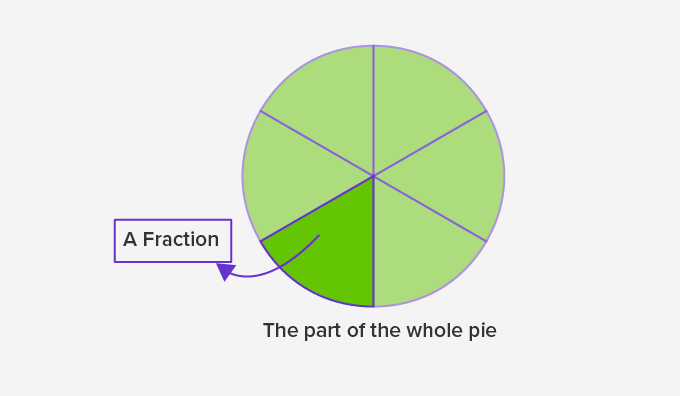A fraction is a part of one whole thing. When a slice of cake is taken out, it becomes a fraction, i.e. a piece of the entire cake.

In fractions, half is written as 12 of the whole.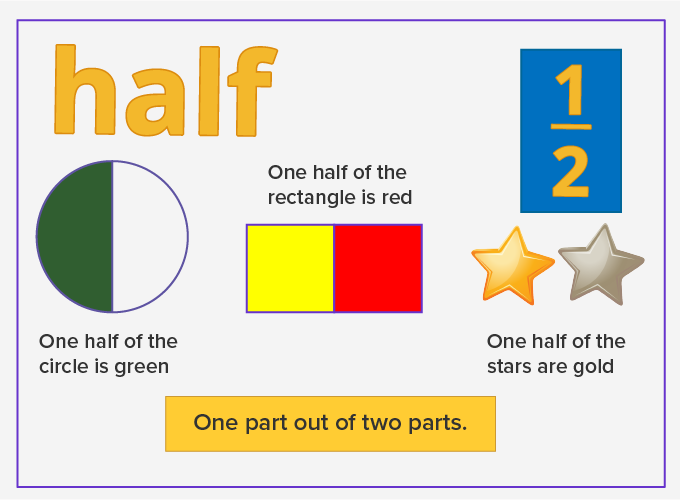Find half of a block comprising of six parts.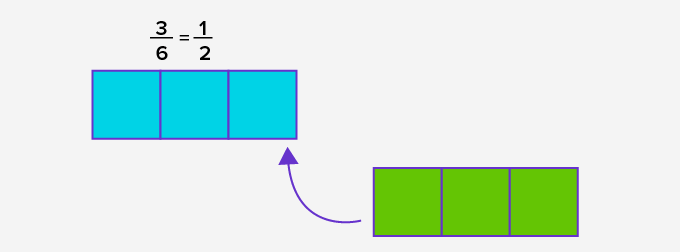Take out 3 parts out of 6, and it equals one half.

Representing half on a number line

We can show fractions on a number line.  The distance from 0-1 on a number line is always one whole. Dividing this distance into two equal parts will give two halves.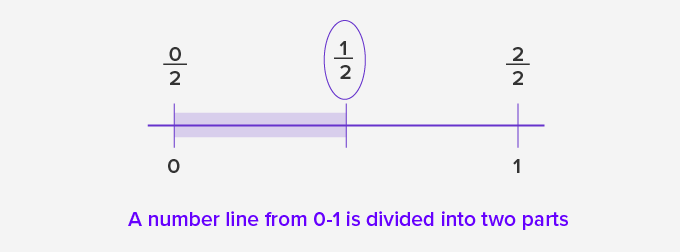## Properties of halves

• Two equal parts of one whole thing.• In decimal numbers, half is denoted by 0.5.

• We can find half of a number multiplying it by ½.  For example, in the figure, half of the group of 6 chips is obtained by 6 × 1=3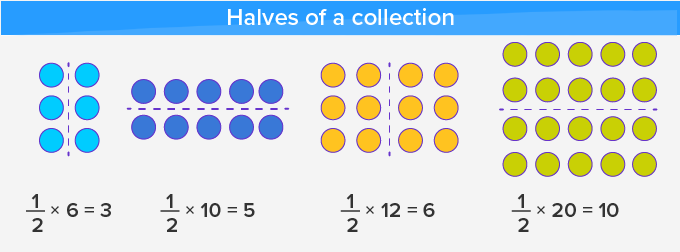• By dividing the number by 2 we can get half of any number.

• Adding two halves makes one.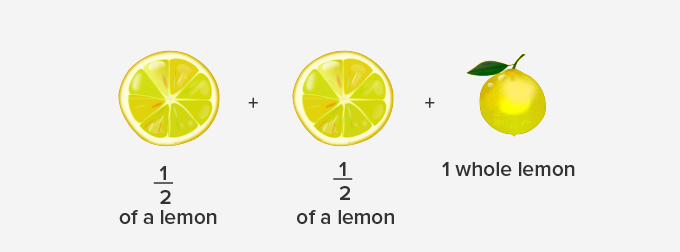Won Numerous Awards & Honors